Win up to 100% scholarship on Aakash BYJU'S JEE/NEET courses with ABNAT Win up to 100% scholarship on Aakash BYJU'S JEE/NEET courses with ABNAT

# JEE Main 2022 June 28 – Shift 2 Chemistry Question Paper with Solutions

JEE Main 2022 June 28 Shift 2 Chemistry question paper with solutions can be accessed here online and in the PDF format. All the JEE main 2022 question papers are solved by our experts to help students verify their answers, and analyse their performance in the examination. Students are advised to solve multiple JEE main question papers to improve their problem-solving skills, and focus on time management in the exam. You can also get the JEE main answer keys for the chemistry papers on our website to verify your answers, whenever you begin to solve the question papers.

## JEE Main 2022 June 28th Chemistry Shift 2 Question Paper and Solutions

#### SECTION – A

Multiple Choice Questions: This section contains 20 multiple choice questions. Each question has 4 choices (1), (2), (3) and (4), out of which ONLY ONE is correct.

1. Compound A contains 8.7% Hydrogen, 74% Carbon and 17.3% Nitrogen. The molecular formula of the compound is,

Given : Atomic masses of C, H and N are 12, 1 and 14 amu, respectively.

The molar mass of the compound A is 162 g mol–1.

(A) C4H6N2

(B) C2H3N

(C) C5H7N

(D) C10H14N2

Sol.

 Element %mass Moles Whole number ratio C 74 6.17 5 H 8.7 8.7 7 N 17.3 1.236 1

Empirical Formula = C5H7N

Empirical formula mass = 81 g

n × 81 = 162

n = 2

Hence, molecular formula is C10H14N2.

2. Consider the following statements :

(A) The principal quantum number ‘n’ is a positive integer with values of ‘n’ = 1, 2, 3, ….

(B) The azimuthal quantum number ‘l’ for a given ‘n’ (principal quantum number) can have values as ‘l’ = 0, 1, 2, …n

(C) Magnetic orbital quantum number ‘ml’ for a particular ‘l’ (azimuthal quantum number) has (2l + 1) values.

(D) ±1/2 are the two possible orientations of electron spin.

(E) For l = 5, there will be a total of 9 orbital

Which of the above statements are correct?

(A) (A), (B) and (C)

(B) (A), (C), (D) and (E)

(C) (A), (C) and (D)

(D) (A), (B), (C) and (D)

Sol. Possible values of l for a given ‘n’ = 0,1,2 …(n – 1)

For l = 5, total orbitals = 2l + 1

= 2(5) + 1 = 11 orbital

Hence A, C and D are correct statements

3. In the structure of SF4, the lone pair of electrons on S is in.

(A) Equatorial position and there are two lone pair – bond pair repulsions at 90º

(B) Equatorial position and there are three lone pair – bond pair repulsions at 90º

(C) Axial position and there are three lone pair – bond pair repulsion at 90º

(D) Axial position and there are two lone pair – bond pair repulsion at 90º

Sol. SF4sp3d hybridisation.The lone pair of electrons on S is in equatorial position and there are two lone pair-bond pair repulsions at 90°.

4. A student needs to prepare a buffer solution of propanoic acid and its sodium salt with pH 4. The ratio of

$$\begin{array}{l}\frac{\left [ CH_3CH_2 COO^- \right ]}{\left [ CH_3CH_2COOH \right ]}\end{array}$$
required to make buffer is _____.

Given : Ka(CH3CH2COOH) = 1.3 × 10–5

(A) 0.03

(B) 0.13

(C) 0.23

(D) 0.33

Sol.

$$\begin{array}{l}CH_3CH_2COOH\rightleftharpoons CH_3CH_2COO^- + H^+\end{array}$$

From Henderson equation

$$\begin{array}{l}pH=pK_a + log\frac{\left [ CH_3CH_2 COO^- \right]}{\left [ CH_3CH_2COOH \right ]}\end{array}$$
$$\begin{array}{l}4 = -\textit{log}~1.3 \times 10^{-5}~ log \frac{\left [ CH_3CH_2 COO^- \right]}{\left [ CH_3CH_2COOH \right ]}\end{array}$$
$$\begin{array}{l}-log 10^{-4}= -log 1.3\times 10^{-5} +log \frac{\left[ CH_3CH_2 COO^- \right]}{\left[ CH_3CH_2COOH \right]}\end{array}$$
$$\begin{array}{l}-log 10^{-4}= -log 1.3\times 10^{-5}\frac{\left [ CH_3CH_2 COOH \right]}{\left [ CH_3CH_2COO^- \right ]}\end{array}$$
$$\begin{array}{l}10^{-4}=1.3\times 10^{-5}\frac{\left [ CH_3CH_2 COOH \right]}{\left [ CH_3CH_2COO^- \right ]}\end{array}$$
$$\begin{array}{l}\frac{\left [ CH_3CH_2 COO^- \right]}{\left [ CH_3CH_2COOH \right ]}= 0.13\end{array}$$

5. Match List-I with List-II :

 List-I List-II (A) Negatively charged sol (I) Fe2O3 . xH2O (B) Macromolecular colloid (II) CdS sol (C) Positively charged sol (III) Starch (D) Cheese (IV) a gel

Choose the correct answer from the options given below:

(A) (A) – (II), (B) – (III), (C) – (IV), (D) – (I)

(B) (A) – (II), (B) – (I), (C) – (III), (D) – (IV)

(C) (A) – (II), (B) – (III), (C) – (I), (D) – (IV)

(D) (A) – (I), (B) – (III), (C) – (II), (D) – (IV)

Sol. (A) Negatively charged sol CdS sol

(B) Macromolecular colloid Starch

(C) Positively charged sol Fe2O3.xH2O

(D) Cheese A gel

6. Match List-I with List-II:

List-I(Oxide) List-II (Nature)

(A) Cl2O7 (I) Amphoteric

(B) Na2O (II) Basic

(C) Al2O3 (III) Neutral

(D) N2O (IV) Acidic

Choose the correct answer from the options given below:

(1) A-IV, B-III, C-I, D-II

(2) A-IV, B-II, C-I, D-III

(3) A-II, B-IV, C-III, D-I

(4) A-I, B-II, C-III, D-IV

Sol. (A) Cl2O7 → Acidic

(B) Na2O → Basic

(C) Al2O3 → Amphoteric

(D) N2O → Neutral

Oxides of metals are basic in nature whereas oxides of non metals are acidic in nature. N2O is a neutral oxide.

7. In the metallurgical extraction of copper, following reaction is used :

FeO + SiO2 → FeSiO3

FeO and FeSiO3 respectively are.

(A) Gangue and flux

(B) Flux and slag

(C) Slag and flux

(D) Gangue and slag

Sol. FeO + SiO2 → FeSiO3

Gangue Slag

8. Hydrogen has three isotopes: protium (1H), deuterium (2H or D) and tritium (3H or T). They have nearly same chemical properties but different physical properties. They differ in

(A) Number of protons

(B) Atomic number

(C) Electronic configuration

(D) Atomic mass

Sol. 1H, 2D and 3H have same atomic number but their atomic masses are different.

Isotopes have same atomic number i.e. same number of protons.

9. Among the following, basic oxide is:

(A) SO3

(B) SiO2

(C) CaO

(D) Al2O3

Sol. Since, oxides of metals are basic in nature. Hence CaO is a basic oxide

SO3 and SiO2 are acidic oxides and Al2O3 is a amphoteric oxide.

10. Among the given oxides of nitrogen; N2O, N2O3, N2O4 and N2O5, the number of compound/(s) having N – N bond is:

(A) 1

(B) 2

(C) 3

(D) 4

Sol.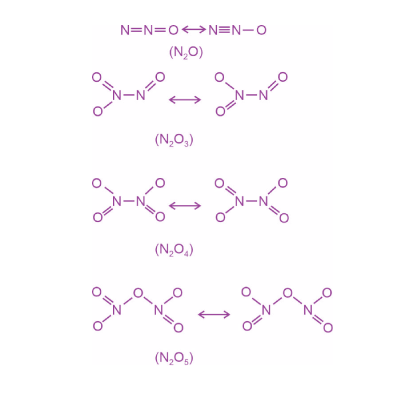N2O, N2O3 and N2O4 contain N — N bond

11. Which of the following oxoacids of sulphur contains ‘‘S’’ in two different oxidation states?

(A) H2S2O3

(B) H2S2O6

(C) H2S2O7

(D) H2S2O8

Sol. In H2S2O3, sulphur exhibits two different oxidation states +6 and –2.12. Correct statement about photo-chemical smog is:

(A) It occurs in humid climate.

(B) It is a mixture of smoke, fog and SO2.

(C) It is reducing smog.

(D) It results from reaction of unsaturated hydrocarbons.

Sol. Photochemical smog occurs in warm, dry and sunny climate. The main components of photochemical smog result from the action of unsaturated hydrocarbons and nitrogen oxides.

This is an oxidising smog.

13. The correct IUPAC name of the following compound is: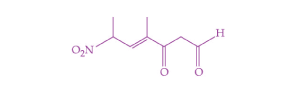(A) 4-methyl-2-nitro-5-oxohept-3-enal

(B) 4-methyl-5-oxo-2-nitrohept-3-enal

(C) 4-methyl-6-nitro-3-oxohept-4-enal

(D) 6-formyl-4-methyl-2-nitrohex-3-enal

Sol.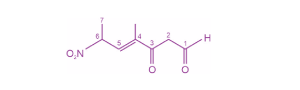4-methyl-6-nitro-3-oxohept-4-enal

14. The major product (P) of the given reaction is (where, Me is –CH3)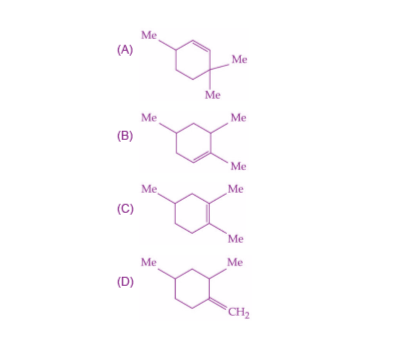Sol.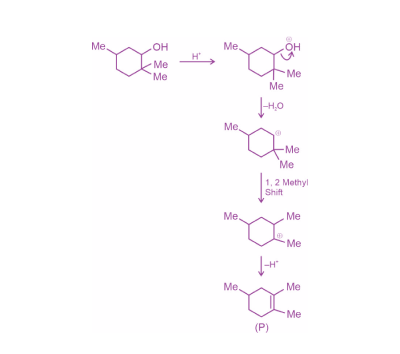15.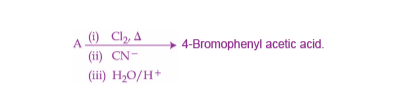In the above reaction ‘A’ is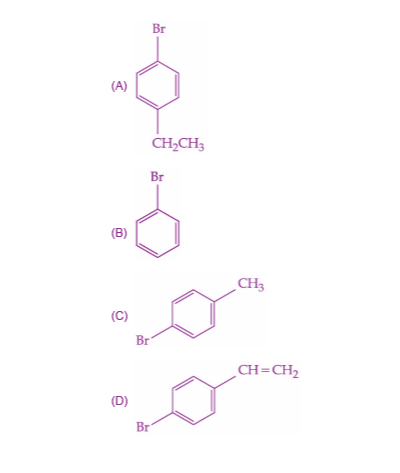Sol.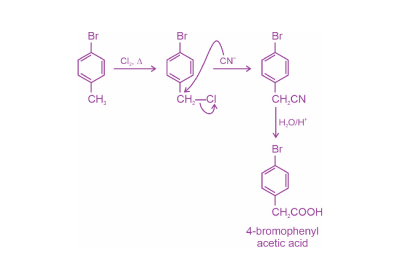16. Isobutyraldehyde on reaction with formaldehyde and K2CO3 gives compound ‘A’. Compound ‘A’ reacts with KCN and yields compound ‘B’, which on hydrolysis gives a stable compound ‘C’. The compound ‘C’ is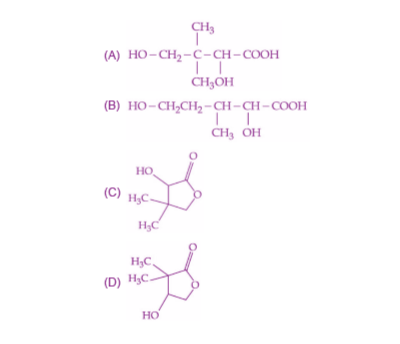Sol.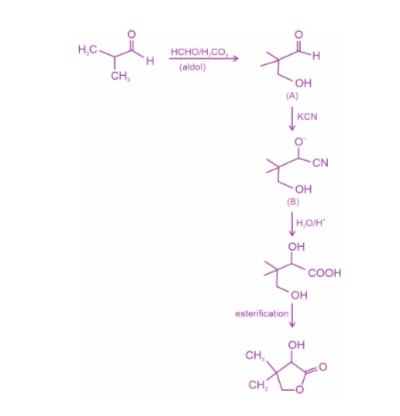17. With respect to the following reaction, consider the given statements: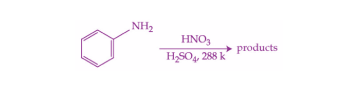(A) o-Nitroaniline and p-nitroaniline are the predominant products.

(B) p-Nitroaniline and m-nitroaniline are the predominant products.

(C) HNO3 acts as an acid.

(D) H2SO4 acts as an acid.

Choose the correct option.

(A) (A) and (C) are correct statements.

(B) (A) and (D) are correct statements.

(C) (B) and (D) are correct statements.

(D) (B) and (C) are correct statements.

Sol.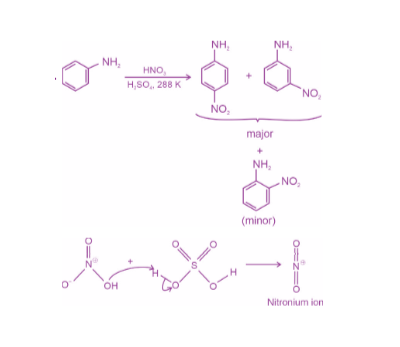Hence, H2SO4 acts as an acid.

18. Given below are two statements, one is Assertion (A) and other is Reason (R).

Assertion (A): Natural rubber is a linear polymer of isoprene called cis-polyisoprene with elastic properties.

Reason (R): The cis-polyisoprene molecules consist of various chains held together by strong polar interactions with coiled structure.

In the light of the above statements, choose the correct one from the options given below:

(A) Both (A) and (R) are true and (R) is the correct explanation of (A).

(B) Both (A) and (R) are true but (R) is not the correct explanation of (A).

(C) (A) is true but (R) is false.

(D) (A) is false but (R) is true.

Sol.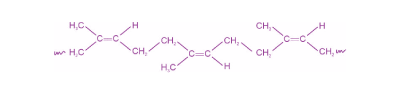The cis-polyisoprene molecule consists of various chains held together by weak van der Waals interactions and has a coiled structure.

Hence assertion is true but reason is false.

19. When sugar ‘X’ is boiled with dilute H2SO4 in alcoholic solution, two isomers ‘A’ and ‘B’ are formed. ‘A’ on oxidation with HNO3 yields saccharic acid whereas ‘B’ is laevorotatory. The compound ‘X’ is :

(A) Maltose

(B) Sucrose

(C) Lactose

(D) Starch

Sol.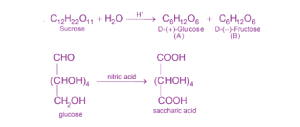D-(–)-Fructose is a laevorotatory compound.

20. The drug tegamet is: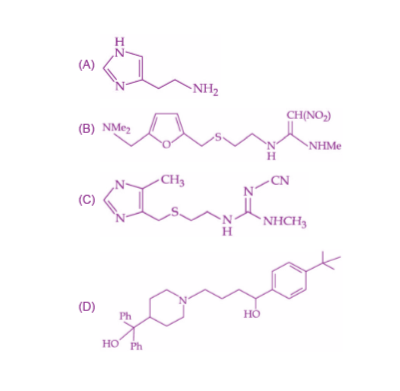Sol. Tegamet (cimetidine) is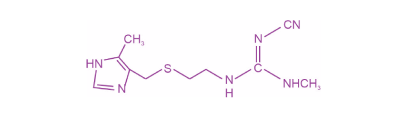#### SECTION – B

Numerical Value Type Questions: This section contains 10 questions. In Section B, attempt any five questions out of 10. The answer to each question is a NUMERICAL VALUE. For each question, enter the correct numerical value (in decimal notation, truncated/rounded-off to the second decimal place; e.g. 06.25, 07.00, –00.33, –00.30, 30.27, –27.30) using the mouse and the on-screen virtual numeric keypad in the place designated to enter the answer.

1. 100 g of an ideal gas is kept in a cylinder of 416 L volume at 27°C under 1.5 bar pressure. The molar mass of the gas is ________ g mol–1. (Nearest integer).

(Given : R = 0.083 L bar K–1 mol–1)

Sol. From combined gas law,

PV = nRT

$$\begin{array}{l}PV = \frac{W}{M}RT\end{array}$$
$$\begin{array}{l}1.5\times 416 = \frac{100}{M}\times 0.083\times 300\end{array}$$

M = 4 g/mol

2. For combustion of one mole of magnesium in an open container at 300 K and 1 bar pressure, ΔCHΘ = –601.70 kJ mol–1, the magnitude of change in internal energy for the reaction is ______ kJ. (Nearest integer)

(Given : R = 8.3 J K–1 mol–1)

Sol.

$$\begin{array}{l}Mg(s)+\frac{1}{2}O_2\rightarrow MgO(s)\end{array}$$
$$\begin{array}{l}\Delta H = \Delta U+\Delta ngRT\end{array}$$
$$\begin{array}{l}\Delta ng = -\frac{1}{2}\end{array}$$
$$\begin{array}{l}-601.70= \Delta U -\frac{1}{2}(8.3)(300)\times 10^{-3}\end{array}$$

ΔU = –601.70 + 1.245

ΔU ≃ –600 kJ

Magnitude of change in internal energy is 600 kJ.

3. 2.5 g of protein containing only glycine (C2H5NO2) is dissolved in water to make 500 mL of solution. The osmotic pressure of this solution at 300 K is found to be 5.03 × 10–3 bar. The total number of glycine units present in the protein is _______.

(Given : R = 0.083 L bar K–1 mol–1)

Sol. Since,

π = icRT

$$\begin{array}{l}5.03 \times 10^{-3}=\frac{2.5}{M} \times \frac{1000}{500}\times 0.083 \times 300\end{array}$$

Molar mass of protein = 24751.5 g/mol

Number of glycine units in protein

$$\begin{array}{l}=\frac{24751.5}{75}\end{array}$$

= 330

4. For the given reactions

Sn2+ + 2e → Sn

Sn4+ + 4e → Sn

the electrode potentials are;

$$\begin{array}{l}E^\circ_{Sn^{2+}/{Sn}} = -0.140 ~\text{V}\ \text{and}\ E^\circ_{Sn^{4+}{Sn}} = -0.010 ~\text{V}.\end{array}$$
The magnitude of standard electrode potential for Sn4+ / Sn2+ i.e.
$$\begin{array}{l}E^\circ_{Sn^{4+}/{Sn^{2+}}}\end{array}$$
is _________ × 10–2 V. (Nearest integer)

Sol.

$$\begin{array}{l}Sn \rightarrow Sn^{2+}+ 2e^- ~~E^\circ_1 =0.140 ~\text{V}\end{array}$$

$$\begin{array}{l}Sn^{4+}+ 4e^- \rightarrow Sn\ \ E^{\circ}_2 =0.010\ \text{V}\end{array}$$
$$\begin{array}{l}Sn^{4+}+ 2e^- \rightarrow Sn^{2+}\ \ E^\circ_{cell} \end{array}$$
$$\begin{array}{l}E^\circ_{cell} = \frac{n_2 E^\circ_2 + n_1E^\circ_1}{n} = \frac{4(0.010)+2(0.140)}{2}\end{array}$$
$$\begin{array}{l}E^\circ_{cell}=0.16~\text{V} = 16 \times 10^{-2} ~\text{V}\end{array}$$

5. A radioactive element has a half life of 200 days. The percentage of original activity remaining after 83 days is ________. (Nearest integer)

(Given : antilog 0.125 = 1.333, antilog 0.693 = 4.93)

Sol.

$$\begin{array}{l}\lambda=\frac{2.303}{t} log \frac{A_0}{A}\end{array}$$
$$\begin{array}{l}\frac{0.693}{200} = \frac{2.303}{83} log \frac{A_0}{A}\end{array}$$
$$\begin{array}{l}\frac{A}{A_0} = 0.75\end{array}$$

Hence, percentage of original activity remaining after 83 days is 75%.

6. [Fe(CN)6]4–

[Fe(CN)6]3–

[Ti(CN)6]3–

[Ni(CN)4]2–

[Co(CN)6]3–

Among the given complexes, number of paramagnetic complexes is_______.

Sol.

 Valence shell configuration Magnetic nature [Fe(CN)6]4– 3d6 (Pairing) Diamagnetic [Fe(CN)6]3– 3d5 (Pairing) Paramagnetic [Ti(CN)6]3– 3d1 Paramagnetic [Ni(CN)4]2– 3d8 (Pairing) Diamagnetic [Co(CN)6]3– 3d6 (Pairing) Diamagnetic

7. (a) CoCl3⋅4 NH3, (b) CoCl3⋅5NH3, (c) CoCl3.6NH3 and (d) CoCl(NO3)2⋅5NH3. Number of complex(es) which will exist in cis-trans form is/are______.

Sol. CoCl3 ⋅ 4NH3 ⇒ [Co(NH3)4Cl2]Cl

CoCl3 ⋅ 5NH3 ⇒ [Co(NH3)5Cl]Cl2

CoCl3 ⋅ 6NH3 ⇒ [Co(NH3)6]Cl3

Only [Co(NH3)4Cl2] can show geometrical isomerism. Hence can exist in cis-trans form.

8. The complete combustion of 0.492 g of an organic compound containing ‘C’, ‘H’ and ‘O’ gives 0.793 g of CO2 and 0.442 g of H2O. The percentage of oxygen composition in the organic compound is_____.[nearest integer]

Sol. CxHyOz + O2 → CO2 + H2O

$$\begin{array}{l}\text{Weight ~of ~carbon}=\frac{0.793}{44}\times 12 = 0.216 ~\text{g}\end{array}$$
$$\begin{array}{l}\text{Weight of hydrogen}=\frac{0.442}{18}\times 2 = 0.05 ~\text{g}\end{array}$$

weight of oxygen = 0.492 – (0.216 + 0.05)

= 0.226 g

$$\begin{array}{l}\text{Percentage by mass of oxygen in compound}=\frac{0.226}{0.492} \times 100\simeq 46\% \end{array}$$

9. The major product of the following reaction contains______bromine atom(s).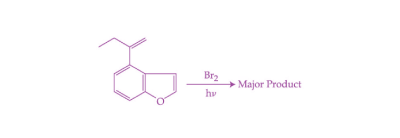Sol.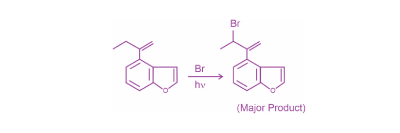10. 0.01 M KMnO4 solution was added to 20.0 mL of 0.05 M Mohr’s salt solution through a burette. The initial reading of 50 mL burette is zero. The volume of KMnO4 solution left in burette after the end point is _____ml. [nearest integer]

Sol. Meq of oxidising agent = Meq of reducing agent

$$\begin{array}{l}(M \times V \times n_F)_{KMnO_4} = (M \times V \times n_F)_{\textup{Mohr’s salt}}\end{array}$$

0.01 × 20 × 5 = 0.05 × V × 1

Volume required = 20 ml

Since initial volume of KMnO4 in burette is 50 ml. Hence volume of KMnO4 left in the burette after end point is 30 ml.

### Download PDF of JEE Main 2022 June 28 Shift 2 Chemistry Paper & Solutions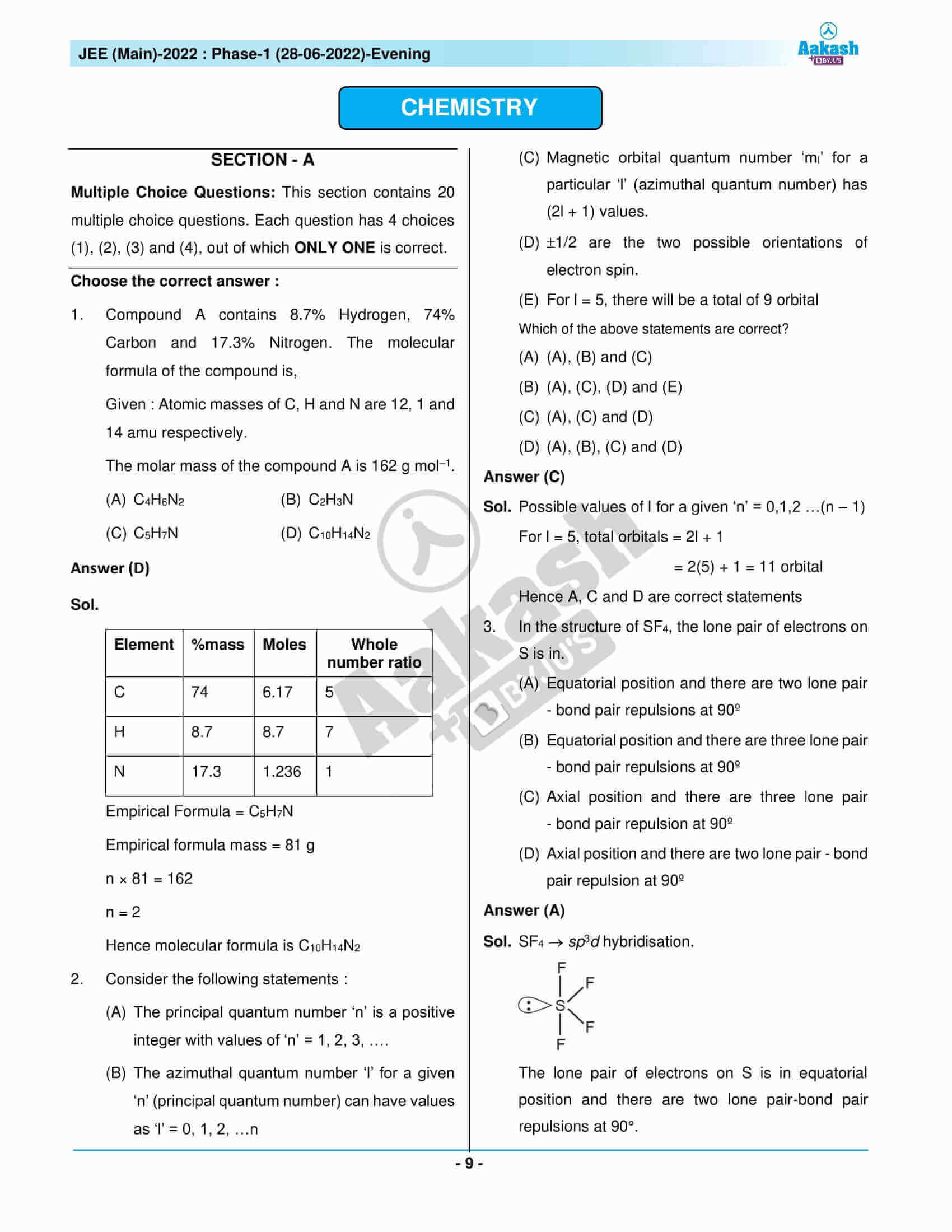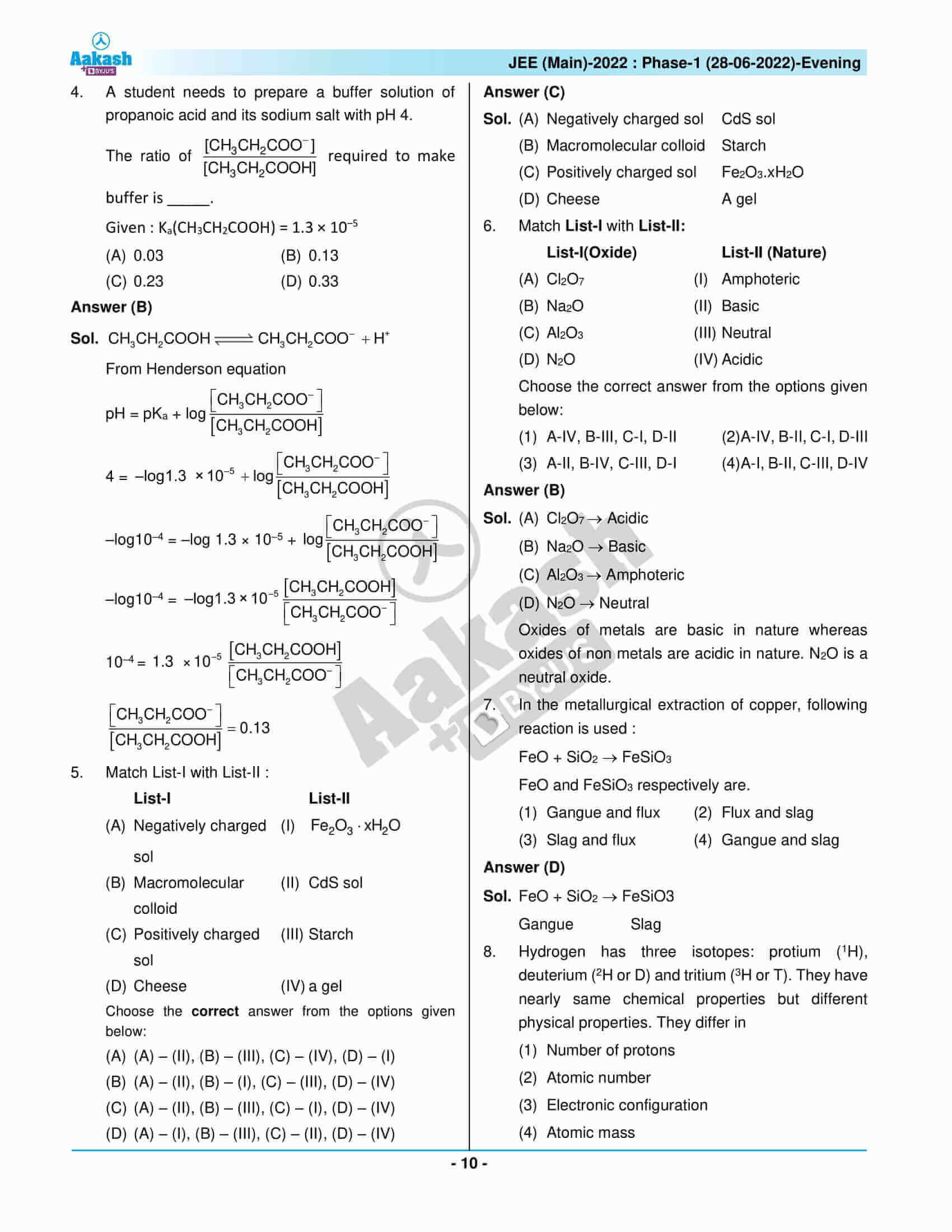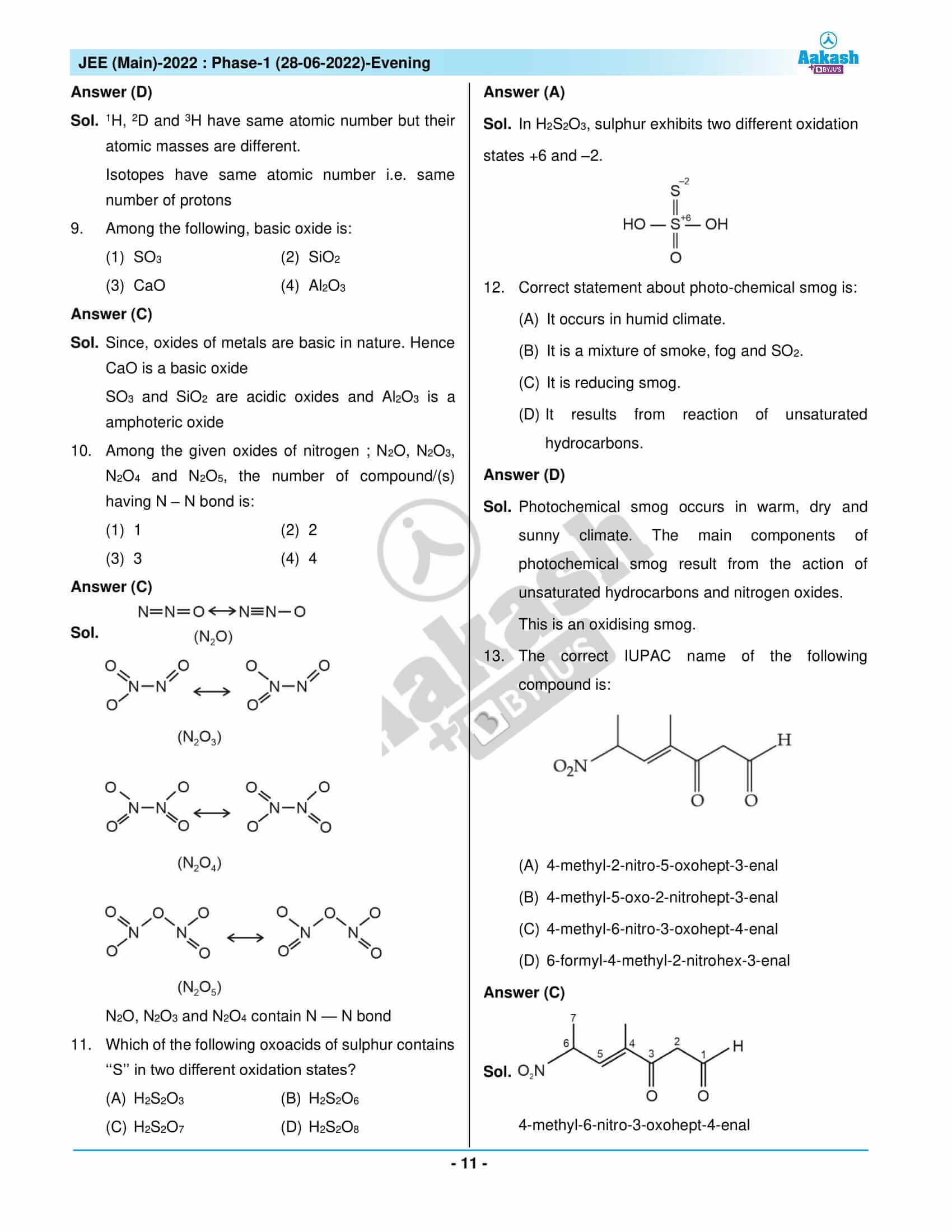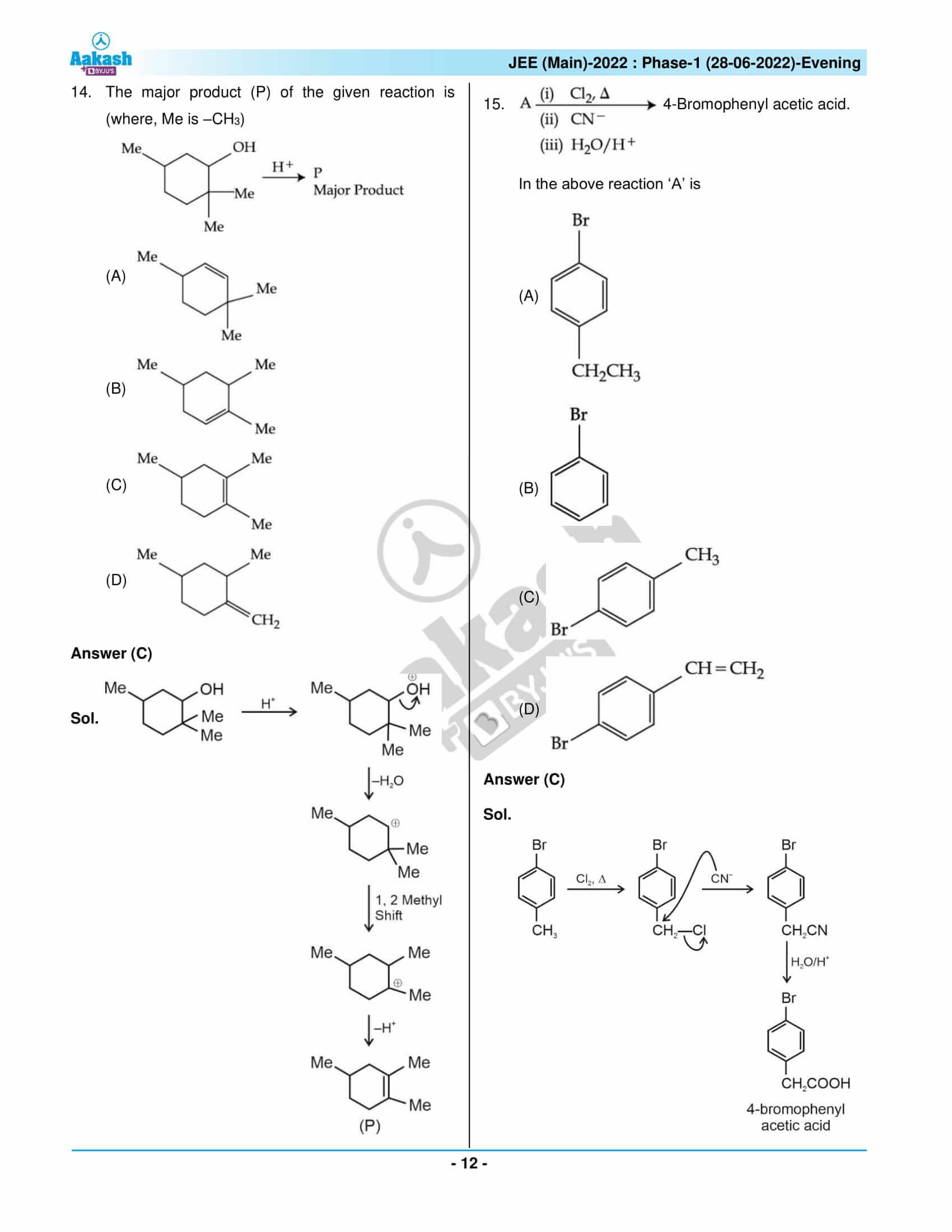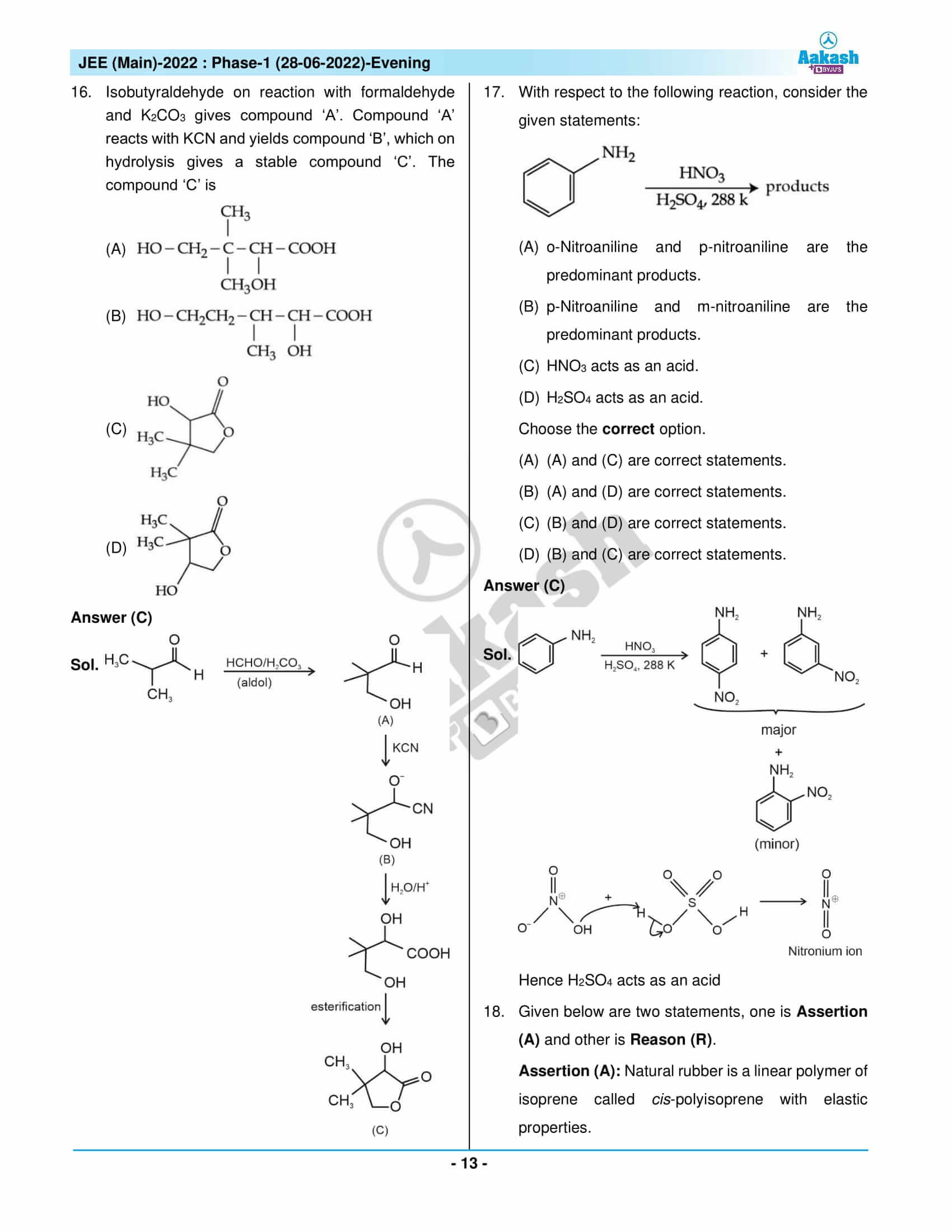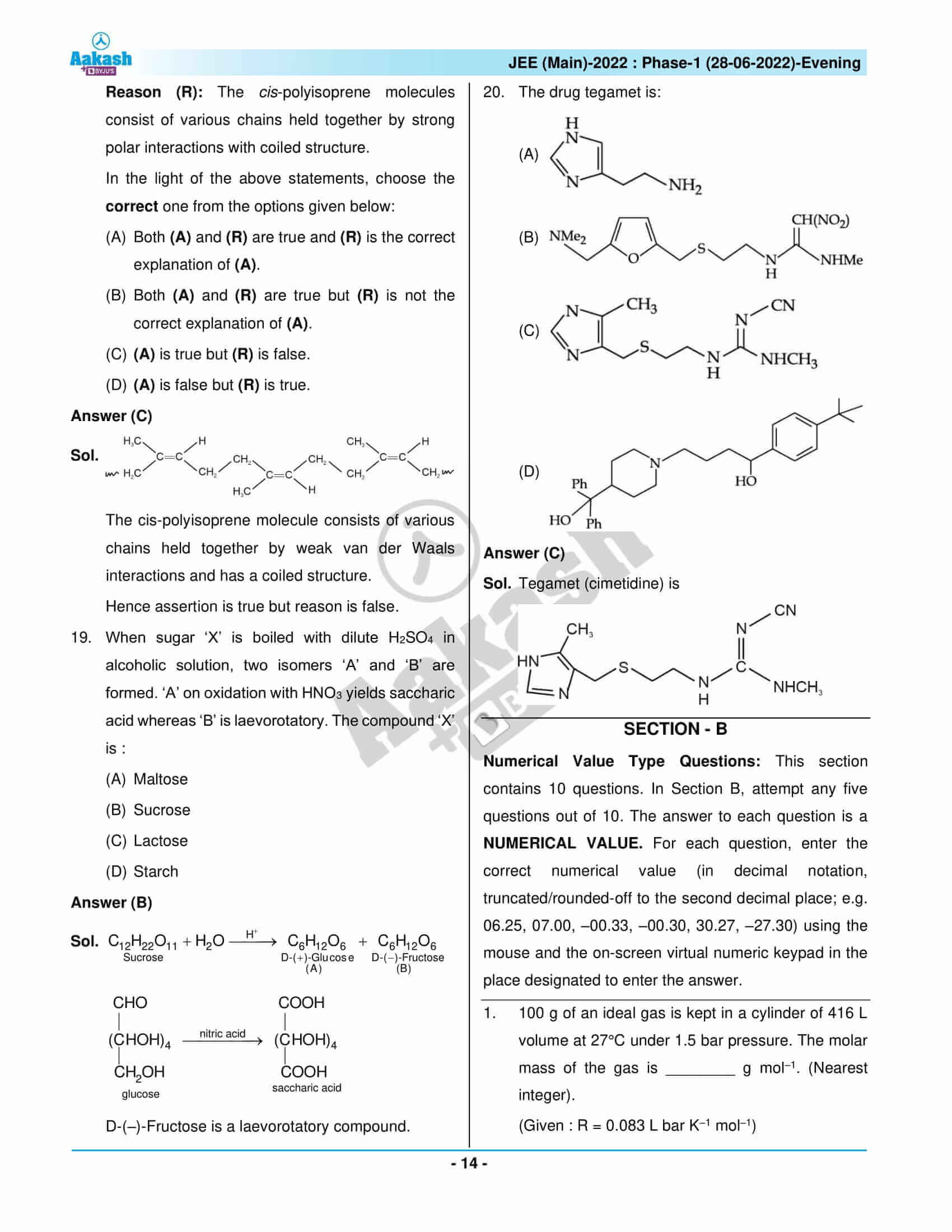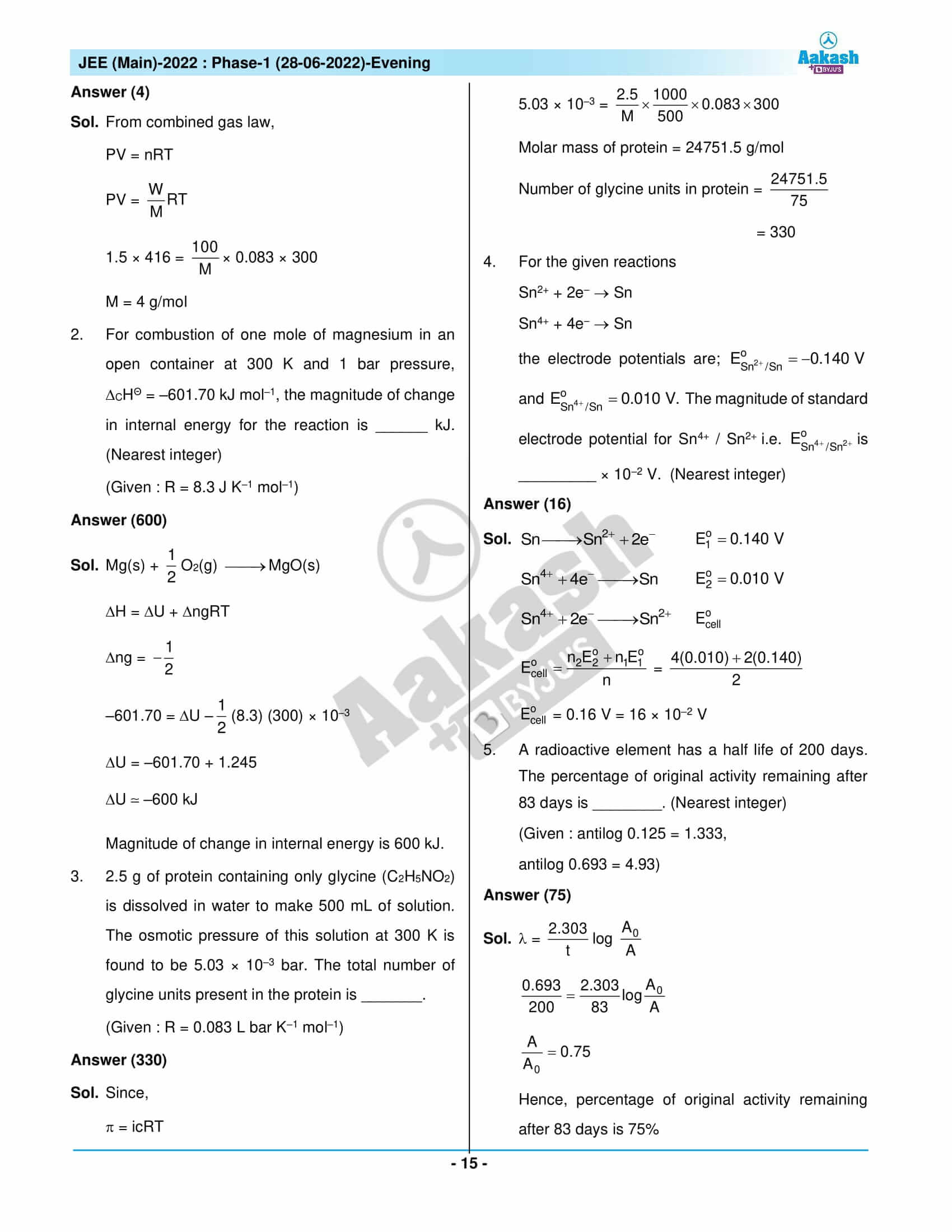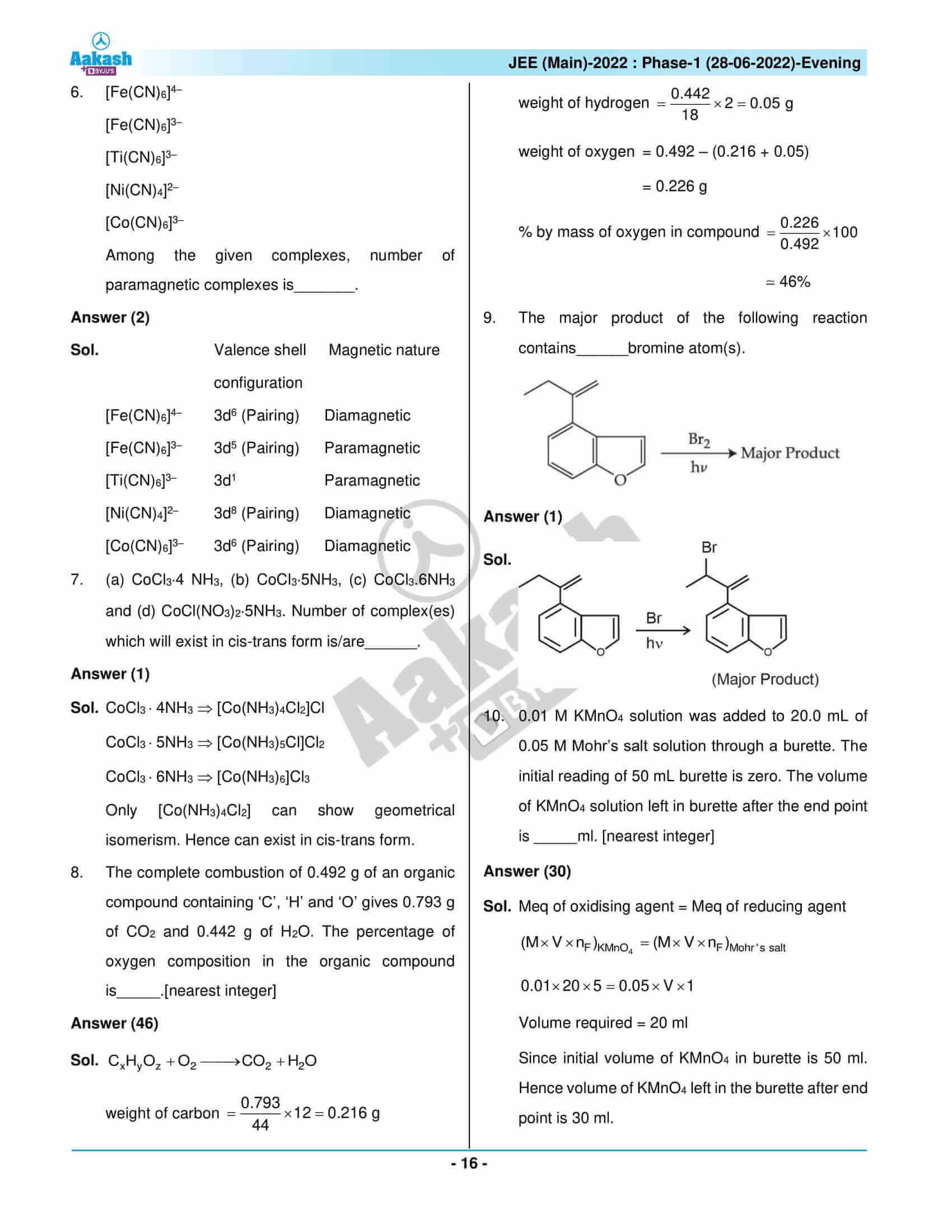## JEE Main 2022 Question Paper with Solutions – June 28 Shift 2

### JEE Main 2022 June 28 Shift 2 Question Paper – Physics Solutions### JEE Main 2022 June 28 Shift 2 Question Paper – Chemistry Solutions### JEE Main 2022 June 28 Shift 2 Question Paper – Maths Solutions## Frequently Asked Questions – FAQs

Q1

### What were the Chemistry chapters from the Class 12 syllabus included in JEE Main 2022 June 28 shift 2 Chemistry question paper?

The Chemistry chapters included from the Class 12 syllabus in JEE Main 2022 June 28 shift 2 Chemistry question paper are:
The P-Block Elements
General Principles and Processes of Isolation of Elements
Organic Chemistry Some Basic Principles and Techniques
Aldehydes, Ketones and Carboxylic Acids
Haloalkanes and Haloarenes
Biomolecules
Surface Chemistry
Chemistry In Everyday Life
Chemical Kinetics and Nuclear Chemistry
Coordination Compounds
Electrochemistry

Q2

### What are topics from which Chemistry questions were included in the JEE Main 2022 June 28 – Shift 2 Question Paper?

The topics from which the questions were asked in the Chemistry section are:
Nitrogen Family
Atmospheric Pollution
Nature of Oxide
Quantum Number
Isotopes of hydrogen
Metallurgy of copper
Nomenclature of Organic Compounds
Compounds of Sulphur
Chemical Reactions of Carboxylic Acids
Empirical Formula
Aromatic Hydrocarbon
Chemical Properties
Disaccharides
Atmospheric Pollution
Buffer Solution
Colloids
The Valence Shell Electron Pair Repulsion (Vsepr) Theory
Therapeutic Action of Different Classes of Drugs
Nuclear Chemistry
Halogenation
Magnetic Properties
Equilibrium Constant and Cell Potential

Q3

### What was the class-wise question distribution of the Chemistry questions in JEE Main 2022 June 28th shift 2 question paper?

Of the 22 memory-based questions, 9 questions were from Class 11 and 13 questions from Class 12.

Q4

### What was the difficulty level of the Chemistry questions in JEE Main 2022 28th June 28 second shift question paper?

The difficulty level of the Chemistry questions was rated as 1.68 on a scale of 1 to 3.

Q5

### What was the nature of the Chemistry questions asked in the JEE Main 2022 June 28 evening shift question paper?

Questions in Chemistry ranged in difficulty from moderate to difficult. Chemistry had 2 difficult questions, 2 easy questions, and 11 medium-level questions.